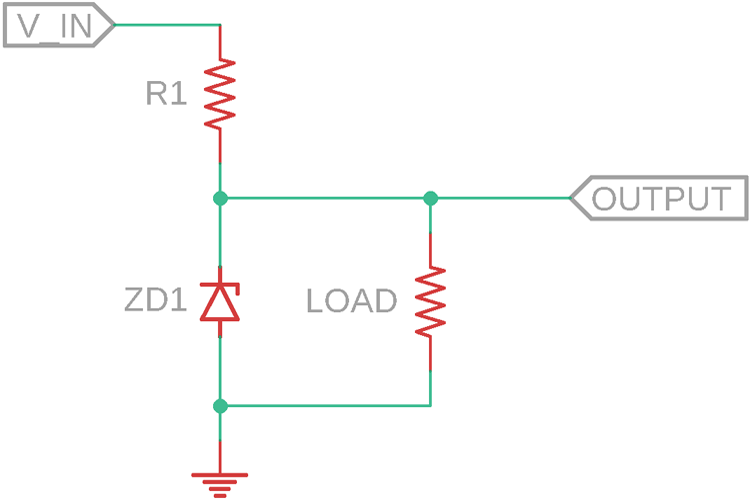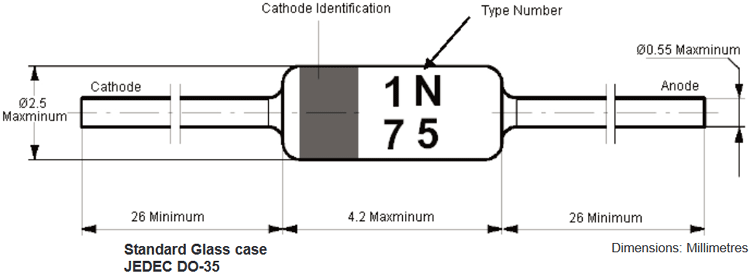# 1N747 Zener Diode

1N747A is a Zener diode from multicomp that offers high reliability, very sharp reverse characteristic, proper voltage stabilization over a wide application in the power electronics field.

This Zener diode offers a 3.6V nominal Zener voltage with ±5% tolerance. It is a 500mW Zener diode that has a forward voltage of 1.5V. It only requires 20mA of bias current to stabilize the regulation.

### 1N747A Pin Description

 Pin Number Pin Name Description 1 Anode Anode pin of the Zener Diode 2 Cathode Cathode pin of the Zener Diode

### Features and Specification of 1N747A Zener Diode

• 500mW power rating
• 3.6V nominal Zener voltage
•  ±5% Zener voltage tolerance
• 20mA bias current required
• DO-35 Package

Note: Complete technical details can be found in the 1N747A Datasheet linked at the bottom of this page.

### Alternative product of 1N747A Zener Diode

Replacement of this 1N747A Zener diode-

1. TZX3V6C
2. 1N5227B
3. MMBZ5227BLT1G

### 1N747A Zener Diode - Overview

1N747A is a 500mW rated 3.6V (VZ) Zener diode. The Zener voltage (VZ) has a tolerance of ±5%. As Zener diode requires biasing current for regulated operations, it requires 20mA of bias current for regulation. The below image is showing the Zener Voltage vs the Zener current graph up to 1 through 16 volts Zener voltage.### 1N747A Zener Diode as a Voltage Regulator

The below image is showing a standard voltage regulator example of Zener diode.The R1 is the biasing resistor for the Zener diode ZD1. It will regulate the output across the Zener diode where the resistive load is connected. However, the above circuit will produce lower efficiency due to unnecessary power dissipation across the biasing resistor R1.

### How to Select Zener Diode

1. Determine the regulation voltage that is required for the application. Choose the Zener voltage as close as the voltage is required. (Vz)

2. Calculate the total load current. Suppose a load requires 100mA of load current, then the actual current of the total circuit will be-

`load current (100mA) + Zener biasing current (5 mA as an example) + 10% or 20% of the load current for safer value (20mA) = Total current (125mA)`

3. The next step is to determine the power dissipation of Zener diode depending on the total current and Zener diode voltage. For example, 1N747A Zener voltage is 3.6V. Thus, in the same parameter, the power dissipation across the Zener diode will be-

` (3.6V x 0.125A) = 0.45W (450mW)`

Choose a Zener diode more than the power rating is actually required. 1N747A has 500mW of power rating.

### Applications of 1N747A Zener Diode

• SMPS
• Overvoltage protection circuit
• Voltage regulators
• Reference voltage generation

### Dimensions of 1N747AComponent Datasheet

## Related Post

### Join 20K+subscribers

We will never spam you.

* indicates required

Be a part of our ever growing community.